# Texas Go Math Grade 4 Lesson 4.3 Answer Key Compare and Order Fractions

Refer to our Texas Go Math Grade 4 Answer Key Pdf to score good marks in the exams. Test yourself by practicing the problems from Texas Go Math Grade 4 Lesson 4.3 Answer Key Compare and Order Fractions.

## Texas Go Math Grade 4 Lesson 4.3 Answer Key Compare and Order Fractions

Essential Question

How can you order fractions?

1. Step 1: Find the least common denominators. Given a set of fractions with unlike denominators, find the least common denominator (LCD) shared by the fractions. …
2. Step 2: Determine the equivalent fractions sharing the LCD. …
3. Step 3: Arrange the numerators. …
4. Step 4: Rewrite the fractions.

Unlock the Problem

• Underline what you need to find.
• Circle the fractions you will compare.

Jody has equal-size bins for the recycling center. She tilled $$\frac{3}{5}$$ of a bin with plastics, $$\frac{1}{12}$$ of a bin with paper, and $$\frac{9}{10}$$ of a bin with glass. Which bin is the most full?
Answer: $$\frac{9}{10}$$
Explanation:
Make common denominator for all
$$\frac{3}{5}$$ = $$\frac{36}{60}$$
$$\frac{1}{12}$$ = $$\frac{5}{60}$$
$$\frac{9}{10}$$ = $$\frac{54}{60}$$

Math Idea
Sometimes it is not reasonable to find the exact location of a point on a number line. Benchmarks can help you find approximate locations.

Example 1

Locate and label $$\frac{3}{5}$$, $$\frac{1}{12}$$, and $$\frac{9}{10}$$ on the number line.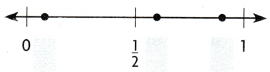STEP 1 Compare each fraction to $$\frac{1}{2}$$.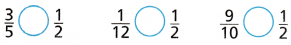___________ and __________ are both greater than 3.
___________ is less than $$\frac{1}{2}$$.
Label $$\frac{1}{12}$$ on the number line above.latex]\frac{3}{5}[/latex] and latex]\frac{9}{10}[/latex] are both greater than 3.
latex]\frac{1}{12}[/latex] is less than $$\frac{1}{2}$$.
Label $$\frac{1}{12}$$ on the number line above.STEP 2 Compare $$\frac{3}{5}$$ and $$\frac{9}{10}$$.
Think: Use 10 as a denominator.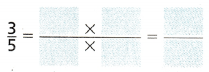Since $$\frac{6}{10}$$ ___________ $$\frac{9}{10}$$, you know that $$\frac{3}{5}$$ ___________ $$\frac{9}{10}$$.
Label $$\frac{3}{5}$$ and $$\frac{9}{10}$$ on the number line above.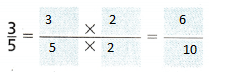Since $$\frac{6}{10}$$ < $$\frac{9}{10}$$, you know that $$\frac{3}{5}$$ <$$\frac{9}{10}$$.
Label $$\frac{3}{5}$$ and $$\frac{9}{10}$$ on the number line above.

The fraction the greatest distance from 0 has the greatest value.
The fraction with the greatest value is ____________ .
So, the bin with ____________ is the most full.

Math Talk

Mathematical Processes
Explain how to write $$\frac{3}{5}$$ and $$\frac{9}{10}$$ as decimals in hundredths and compare their distances from 0.
$$\frac{3}{5}$$ = $$\frac{60}{100}$$
$$\frac{9}{10}$$ = $$\frac{90}{100}$$

Explain how to write $$\frac{3}{5}$$ and $$\frac{9}{10}$$ as decimals in tenths and compare their distances from 0.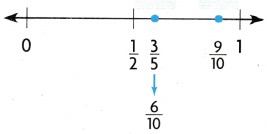Example 2

Write $$\frac{7}{10}$$, $$\frac{1}{3}$$, $$\frac{7}{12}$$, and $$\frac{8}{10}$$ in order from least to greatest.Step 1 Compare each fraction to $$\frac{1}{2}$$.
List fractions that are less than $$\frac{1}{2}$$: ________________
List fractions that are greater than $$\frac{1}{2}$$: ________________
The fraction with the least value is ________________.
Locate and label $$\frac{1}{3}$$ on the number tine above.

Step 2 Compare $$\frac{7}{10}$$ to $$\frac{7}{12}$$ and $$\frac{8}{10}$$.

Think: $$\frac{7}{10}$$ and $$\frac{7}{12}$$ have equal numerators.
$$\frac{7}{10}$$ ___________ $$\frac{7}{12}$$

Think: $$\frac{7}{10}$$ and $$\frac{8}{10}$$ have equal denominators.
$$\frac{7}{10}$$ ___________ $$\frac{8}{10}$$

Locate and label $$\frac{7}{10}$$, $$\frac{7}{12}$$, and $$\frac{8}{10}$$ on the number line above.
The fractions in order from least to greatest are ___________ .
So, _____ < _____ < _____ < ______ .

Math Talk

Mathematical Processes

Share and Show

Question 1.
Locate and label points on the number line to help you write $$\frac{3}{10}$$, $$\frac{11}{12}$$, and $$\frac{5}{8}$$ in order from least to greatest.Explanation:
Make the common denominator for all
$$\frac{3}{10}$$= $$\frac{36}{120}$$
$$\frac{11}{12}$$= $$\frac{110}{120}$$
$$\frac{5}{8}$$ = $$\frac{75}{120}$$

Write the fractions in order from least to greatest.

Lesson 4.3 Problem Set Answer Key Go Math Grade 4 Question 2.
$$\frac{1}{4}, \frac{5}{8}, \frac{1}{2}$$
Answer: $$\frac{1}{4}, \frac{1}{2}, \frac{5}{8}$$
Explanation:
Make the common denominator for all
$$\frac{1}{4}$$ = $$\frac{2}{8}$$
$$\frac{5}{8}$$ = $$\frac{5}{8}$$
$$\frac{1}{2}$$ = $$\frac{4}{8}$$

Question 3.
$$\frac{3}{5}, \frac{2}{3}, \frac{3}{10}, \frac{4}{5}$$
Answer: $$\frac{3}{10},\frac{3}{5}, \frac{2}{3}, \frac{4}{5}$$
Explanation:
Make the common denominator for all
$$\frac{3}{5}$$ = $$\frac{18}{30}$$
$$\frac{2}{3}$$ = $$\frac{20}{30}$$
$$\frac{3}{10}$$ = $$\frac{9}30}$$
$$\frac{4}{5}$$ = $$\frac{24}{30}$$

Question 4.
$$\frac{3}{4}, \frac{7}{12}, \frac{5}{12}$$
Answer: $$\frac{5}{12},\frac{7}{12}, \frac{3}{4}$$
Explanation:
Make the common denominator for all
$$\frac{3}{4}$$ = $$\frac{9}{12}$$
$$\frac{7}{12}$$ = $$\frac{7}{12}$$
$$\frac{5}{12}$$ = $$\frac{5}{12}$$

H.O.T. Algebra Write a numerator that makes the statement true.

Question 5.
$$\frac{1}{2}$$ < $$\frac{}{10}$$ < $$\frac{4}{5}$$
Answer: $$\frac{1}{2}$$ < $$\frac{6}{10}$$ < $$\frac{4}{5}$$
Explanation:
Make the common denominator for all
$$\frac{1}{2}$$ = $$\frac{5}{10}$$
$$\frac{5}{10}$$ = $$\frac{}{10}$$
$$\frac{4}{5}$$ = $$\frac{8}{10}$$

Question 6.
$$\frac{1}{4}$$ < $$\frac{5}{12}$$ < $$\frac{}{6}$$
Answer: $$\frac{1}{4}$$ < $$\frac{5}{12}$$ < $$\frac{1}{6}$$
Explanation:
Make the common denominator for all
$$\frac{1}{4}$$ = $$\frac{3}{12}$$
$$\frac{5}{12}$$ = $$\frac{5}{12}$$
$$\frac{}{6}$$ = $$\frac{6}{12}$$ = $$\frac{1}{6}$$

$$\frac{}{8}$$ < $$\frac{3}{4}$$ < $$\frac{7}{8}$$
Answer: $$\frac{5}{8}$$ < $$\frac{3}{4}$$ < $$\frac{7}{8}$$
Explanation:
Make the common denominator for all
$$\frac{}{8}$$ = $$\frac{5}{8}$$
$$\frac{3}{4}$$ = $$\frac{6}{8}$$
$$\frac{7}{8}$$ = $$\frac{7}{8}$$

Problem Solving

Question 8.
H.O.T. Multi-Step Nancy, Lionel, and Mavis ran in a 5-kilometer race. The table shows their finish times. In what order did Nancy, Lionel, and Mavis finish the race?a. What do you need to find?

b. What information do you need to solve the problem?

c. What information is not necessary?

d. How will you solve the problem?

e. Show the steps to solve the problem.

f. Complete the sentences.
The runner who finished first is _________ .
The runner who finished second is _________ .
The runner who finished third is __________ .

Question 9.
Multi-Step Alma used 3 beads to make a necklace. The lengths of the beads are $$\frac{5}{6}$$ inch, $$\frac{5}{12}$$ inch, and $$\frac{1}{3}$$ inch. What are the lengths in order from shortest to longest?
Answer: $$\frac{1}{3}$$ , $$\frac{5}{12}$$, $$\frac{5}{6}$$
Explanation:
Make the common denominator for all
$$\frac{5}{6}$$ = $$\frac{10}{12}$$
$$\frac{5}{12}$$ = $$\frac{5}{12}$$
$$\frac{1}{3}$$ = $$\frac{4}{12}$$

Question 10.
H.O.T. Apply Portia lias done $$\frac{3}{7}$$ of her English homework, $$\frac{6}{7}$$ of her math homework, and $$\frac{6}{11}$$ of her geography homework. Which subject is most complete? Which subject does she have the most left to do?
Answer: Math subject is most completed. English subject most left .
Explanation:
Make the common denominator for all
$$\frac{3}{7}$$ = $$\frac{33}{77}$$
$$\frac{6}{7}$$ = $$\frac{66}{77}$$
$$\frac{6}{11}$$ = $$\frac{42}{77}$$

Question 11.
Use Diagrams Students voted for their favorite hat on Crazy Hat Day. An alien baseball cap got $$\frac{1}{4}$$ of the votes, a flower power hat got $$\frac{5}{8}$$ of the votes, and an animal look-alike hat got $$\frac{1}{12}$$ of the votes. Which shows the fractions written in order from least to greatest?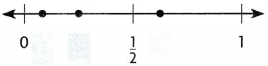(A) $$\frac{1}{12}$$ < $$\frac{5}{8}$$ < $$\frac{1}{4}$$
(B) $$\frac{1}{12}$$ < $$\frac{1}{4}$$ < $$\frac{5}{8}$$
(C) $$\frac{5}{8}$$ < $$\frac{1}{4}$$ < $$\frac{1}{12}$$
(D) $$\frac{1}{4}$$ < $$\frac{1}{12}$$ < $$\frac{5}{8}$$
Explanation:
Make the common denominator for all
$$\frac{1}{4}$$ = $$\frac{6}{24}$$
$$\frac{5}{8}$$ = $$\frac{15}{24}$$
$$\frac{1}{12}$$ = $$\frac{2}{24}$$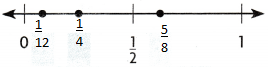Multi-Step The fourth grade gym class ran a relay. The first runner ran $$\frac{4}{5}$$ mile, the second runner ran $$\frac{3}{10}$$ mile, the third runner ran $$\frac{1}{5}$$ mile and the fourth runner ran $$\frac{7}{10}$$ mile Compare $$\frac{4}{5}, \frac{3}{10}, \frac{1}{5}$$, and $$\frac{7}{10}$$. Which fraction represents the greatest distance?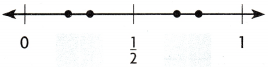(A) $$\frac{4}{5}$$
(B) $$\frac{3}{10}$$
(C) $$\frac{1}{5}$$
(D) $$\frac{7}{10}$$
Answer: $$\frac{4}{5}$$
Explanation:
Make the common denominator for all
$$\frac{4}{5}$$ = $$\frac{8}{10}$$
$$\frac{3}{10}$$ = $$\frac{3}{10}$$
$$\frac{1}{5}$$ = $$\frac{2}10}$$
$$\frac{7}{10}$$ = $$\frac{7}{10}$$Question 13.
Multi-Step The three puppies at the animal shelter weighed $$\frac{2}{3}$$ pound, $$\frac{5}{6}$$ pound, and $$\frac{7}{12}$$ pound. Compare $$\frac{2}{3}$$, $$\frac{5}{6}$$, and $$\frac{7}{12}$$. Which shows the fractions written in order from least to greatest?
(A) $$\frac{2}{3}$$ < $$\frac{7}{12}$$ < $$\frac{5}{6}$$
(B) $$\frac{2}{3}$$ < $$\frac{5}{6}$$ < $$\frac{7}{12}$$
(C) $$\frac{7}{12}$$ < $$\frac{5}{6}$$ < $$\frac{2}{3}$$
(D) $$\frac{7}{12}$$ < $$\frac{2}{3}$$ < $$\frac{5}{6}$$
Answer: $$\frac{7}{12}$$ < $$\frac{2}{3}$$ < $$\frac{5}{6}$$
Make the common denominator for all
$$\frac{2}{3}$$ = $$\frac{8}{12}$$
$$\frac{5}{6}$$ = $$\frac{10}{12}$$
$$\frac{7}{12}$$ = $$\frac{7}{12}$$

TEXAS Test Prep

Question 14.
A recipe for Trail Mix includes $$\frac{3}{10}$$ cup of sunflower seeds, $$\frac{1}{2}$$ cup of raisins, and $$\frac{3}{8}$$ cup of granola. Which list shows the amounts from least to greatest?
(A) $$\frac{1}{2}$$cup, $$\frac{3}{8}$$cup, $$\frac{3}{10}$$cup
(B) $$\frac{3}{8}$$cup, $$\frac{3}{10}$$cup, $$\frac{1}{2}$$cup
(C) $$\frac{3}{10}$$cup, $$\frac{3}{8}$$cup, $$\frac{1}{2}$$cup
(D) $$\frac{3}{10}$$cup, $$\frac{1}{2}$$cup, $$\frac{3}{8}$$cup
Answer: $$\frac{2}{8},\frac{2}{6}, \frac{2}{4}$$
Explanation: (C)   $$\frac{3}{10}$$cup, $$\frac{3}{8}$$cup, $$\frac{1}{2}$$cup
Make the common denominator for all
$$\frac{3}{10}$$ = $$\frac{12}{40}$$
$$\frac{1}{2}$$ = $$\frac{20}{40}$$
$$\frac{3}{8}$$ = $$\frac{15}{40}$$

### Texas Go Math Grade 4 Lesson 4.3 Homework and Practice Answer Key

Write the fractions in order from least to greatest.

Question 1.
$$\frac{2}{8}, \frac{2}{4}, \frac{2}{6}$$
Answer: $$\frac{2}{8},\frac{2}{6}, \frac{2}{4}$$
Explanation:
Make the common denominator for all
$$\frac{2}{8}$$ = $$\frac{6}{24}$$
$$\frac{2}{4}$$ = $$\frac{12}{24}$$
$$\frac{2}{6}$$ = $$\frac{8}{24}$$

Question 2.
$$\frac{2}{5}, \frac{1}{3}, \frac{5}{6}$$
Answer: $$\frac{1}{3},\frac{2}{5}, \frac{5}{6}$$
Explanation:
Make the common denominator for all
$$\frac{2}{5}$$ = $$\frac{12}{30}$$
$$\frac{1}{3}$$ = $$\frac{10}{30}$$
$$\frac{5}{6}$$ = $$\frac{25}{30}$$

Write a numerator that makes the statement true.

Question 3.
$$\frac{7}{12}$$ < $$\frac{}{3}$$ < $$\frac{3}{4}$$
Answer: $$\frac{7}{12}$$ < $$\frac{2}{3}$$ < $$\frac{3}{4}$$
Explanation:
Make the common denominator for all
$$\frac{7}{12}$$ = $$\frac{7}{12}$$
$$\frac{}{3}$$ = $$\frac{8}{12}$$  = latex]\frac{2}{3}[/latex]
$$\frac{3}{4}$$ = $$\frac{9}{12}$$

Go Math Grade 4 Lesson 4.3 Practice and Homework Answer Key Question 4.
$$\frac{}{10}$$ < $$\frac{9}{15}$$ < $$\frac{4}{5}$$
Answer: $$\frac{5}{10}$$ < $$\frac{9}{15}$$ < $$\frac{4}{5}$$
Explanation:
Make the common denominator for all
$$\frac{}{10}$$ = $$\frac{15}{30}$$ =$$\frac{5}{10}$$
$$\frac{9}{15}$$ = $$\frac{18}{30}$$
$$\frac{4}{5}$$ = $$\frac{24}{30}$$

Problem Solving

Question 5.
Walt, Dalia, and Kyra ran a race. The table shows their finish times. In what order did Walt, Dalia, and Kyra finish the race?Answer: Paul, Dalia , Walt , Kyra
Explanation:
Walt – $$\frac{4}{5}$$ = 0.8 hour = 48 min
Dalia –$$\frac{2}{3}$$ = 0.66 hour =39.6 min
Kyra – $$\frac{5}{6}$$ = 0.83 hour = 49.8 min
Paul- $$\frac{3}{10}$$ = 0.3 hour = 18 min

Question 6.
Walt’s friend Paul also ran in the race. Who finished first, Walt or Paul?
Explanation:
Walt – $$\frac{4}{5}$$ = 0.8 hour = 48 min
Dalia –$$\frac{2}{3}$$ = 0.66 hour =39.6 min
Kyra – $$\frac{5}{6}$$ = 0.83 hour = 49.8 min
Paul- $$\frac{3}{10}$$ = 0.3 hour = 18 min

Lesson Check

Question 7.
A recipe for ice cream includes $$\frac{3}{4}$$ cup milk, $$\frac{1}{3}$$ cup cream, and $$\frac{1}{8}$$ cup sugar. Which shows the amounts from least to greatest?
(A) $$\frac{1}{3}$$cup, $$\frac{3}{4}$$cup, $$\frac{1}{8}$$cup
(B) $$\frac{1}{8}$$cup, $$\frac{3}{4}$$cup, $$\frac{1}{8}$$cup
(C) $$\frac{1}{3}$$cup, $$\frac{3}{4}$$cup, $$\frac{1}{8}$$cup
(D) $$\frac{1}{8}$$cup, $$\frac{1}{3}$$cup,$$\frac{3}{4}$$cup
Explanation:
$$\frac{1}{3}$$ = 0.33
$$\frac{3}{4}$$ = 0.75
$$\frac{1}{8}$$ =  0.12

Question 8.
Order the fractions from least to greatest.
$$\frac{4}{5}, \frac{1}{3}, \frac{7}{10}, \frac{3}{5}$$
(A) $$\frac{1}{3}$$ < $$\frac{3}{5}$$ < $$\frac{7}{10}$$ < $$\frac{4}{5}$$
(B) $$\frac{3}{5}$$ < $$\frac{1}{3}$$ < $$\frac{4}{5}$$ < $$\frac{7}{10}$$
(C) $$\frac{7}{10}$$ < $$\frac{3}{5}$$ < $$\frac{1}{3}$$ < $$\frac{4}{5}$$
(D) $$\frac{4}{5}$$ < $$\frac{7}{10}$$ < $$\frac{1}{3}$$ < $$\frac{3}{5}$$
Explanation:
$$\frac{4}{5}$$ =0.8
$$\frac{7}{10}$$ =0.7
$$\frac{1}{3}$$ = 0.33
$$\frac{3}{5}$$ = 0.6
Based on above decimal value , below is the order from least to greatest
$$\frac{1}{3}$$ < $$\frac{3}{5}$$ < $$\frac{7}{10}$$ < $$\frac{4}{5}$$

Question 9.
Order the fractions from least to greatest.
$$\frac{2}{3}, \frac{1}{4}, \frac{5}{12}, \frac{3}{4}$$
(A) $$\frac{1}{4}, \frac{2}{3}, \frac{5}{12}, \frac{3}{4}$$
(B) $$\frac{3}{4}, \frac{5}{12}, \frac{2}{3}, \frac{1}{4}$$
(C) $$\frac{1}{4}, \frac{5}{12}, \frac{2}{3}, \frac{3}{4}$$
(D) $$\frac{1}{4}, \frac{5}{12}, \frac{3}{4}, \frac{2}{3}$$
Answer: (D) $$\frac{1}{4}, \frac{5}{12}, \frac{3}{4}, \frac{2}{3}$$
Explanation:
$$\frac{2}{3}$$ =0.66
$$\frac{1}{4}$$ =0.25
$$\frac{5}{12}$$ = 0.41
$$\frac{3}{4}$$ = 0.75
below is the Order the fractions from least to greatest.
$$\frac{1}{4}, \frac{5}{12}, \frac{3}{4}, \frac{2}{3}$$

Question 10.
Three potatoes weigh $$\frac{1}{4}$$ pound, $$\frac{5}{8}$$ pound, and $$\frac{1}{2}$$ pound. Which shows the weights from least to greatest?
(A) $$\frac{1}{4}$$pound, $$\frac{5}{8}$$pound, $$\frac{1}{2}$$pound
(B) $$\frac{1}{2}$$pound, $$\frac{1}{4}$$pound, $$\frac{5}{8}$$pound
(C) $$\frac{5}{8}$$pound, $$\frac{1}{2}$$pound, $$\frac{1}{4}$$pound
(D) $$\frac{1}{4}$$pound, $$\frac{1}{2}$$pound, $$\frac{5}{8}$$pound
Answer: (D) $$\frac{1}{4}$$pound, $$\frac{1}{2}$$pound, $$\frac{5}{8}$$pound
Explanation:
$$\frac{1}{4}$$ = 0.25
$$\frac{5}{8}$$ = 0.625
$$\frac{1}{2}$$ = 0.5
Based on the above decimal values of weights, below is the order from least to greatest
$$\frac{1}{4}$$pound, $$\frac{1}{2}$$pound, $$\frac{5}{8}$$pound

My Homework Lesson 4.3 Compare and Order Fractions Answer Key Question 11.
Multi-Step Selma used stones to outline her garden. The lengths of the stones are $$\frac{1}{3}$$foot, $$\frac{7}{12}$$ foot, and $$\frac{3}{4}$$ foot. What are the lengths in order from shortest to longest?
(A) $$\frac{7}{12}$$foot, $$\frac{3}{4}$$foot, $$\frac{1}{3}$$foot
(B) $$\frac{1}{3}$$foot, $$\frac{7}{12}$$foot, $$\frac{3}{4}$$foot
(C) $$\frac{3}{4}$$foot, $$\frac{7}{12}$$foot, $$\frac{1}{3}$$foot
(D) $$\frac{7}{12}$$foot, $$\frac{1}{3}$$foot, $$\frac{3}{4}$$foot
Answer: (B) $$\frac{1}{3}$$foot, $$\frac{7}{12}$$foot, $$\frac{3}{4}$$foot
Explanation:
$$\frac{7}{12}$$ =0.58
$$\frac{3}{4}$$ = 0.75
$$\frac{1}{3}$$ = 0.33
Based on above decimal values of lengths, below is the order from shortest to longest
$$\frac{1}{3}$$foot, $$\frac{7}{12}$$foot, $$\frac{3}{4}$$foot

Question 12.
Multi-Step Ms. Mohan bought cheese for a recipe. She bought $$\frac{5}{6}$$ pound of cheddar cheese, $$\frac{1}{4}$$ pound of Swiss cheese, and $$\frac{3}{8}$$ pound of American cheese. What are the amounts in order from least to greatest?
(A) $$\frac{5}{6}$$pound, $$\frac{1}{4}$$pound, $$\frac{3}{8}$$pound
(B) $$\frac{3}{8}$$pound, $$\frac{5}{6}$$pound, $$\frac{1}{4}$$pound
(C) $$\frac{1}{4}$$pound, $$\frac{3}{8}$$pound, $$\frac{5}{6}$$pound
(D) $$\frac{3}{8}$$pound, $$\frac{1}{4}$$pound, $$\frac{5}{6}$$pound
Answer: (C) $$\frac{1}{4}$$pound, $$\frac{3}{8}$$pound, $$\frac{5}{6}$$pound
Explanation:
$$\frac{5}{6}$$= 0.83
$$\frac{1}{4}$$ = 0.25
$$\frac{3}{8}$$ = 0.37
Based on above decimal values, below is the order from least to greatest
$$\frac{1}{4}$$pound, $$\frac{3}{8}$$pound, $$\frac{5}{6}$$pound

Scroll to Top